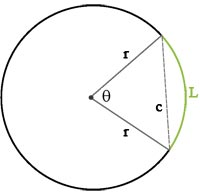# Arc of a Circle Calculator

Whether you need to calculate the length of an arc, chord length, or other properties of a circle, our calculator is here to make your life easier.

How to use the Calculator:

1. Enter the diameter to calculate the radius or input the radius to find the diameter.
2. Enter either the angle of the arc or the length of the arc.

By performing these operations, you can quickly find the Arc of a Circle.

But that's not all! Our Arc of a Circle Calculator can also help you:

• Find the radius of a circle, knowing only the diameter.
• Estimate the diameter of a circle when its radius is known.
• Find the length of an arc, using the chord length and arc angle.
• Compute the arc angle by inserting the values of the arc length and radius.
Arc of a Circle Calculator°

## Formulas

This calculator uses the following formulas: